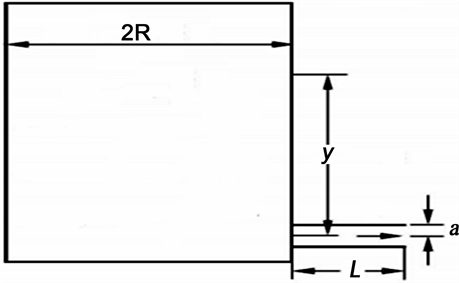﻿ 液体粘滞系数测量实验中的几点思考

# 液体粘滞系数测量实验中的几点思考Suggestions on the Measurement of Liquid Viscosity Coefficient

Abstract: In this paper, we analyzed water viscosity coefficient through exponential fitting and linear fitting in the experiment of measuring by capillary method in engineering colleges. In addition, the higher the timing start height, the closer to the theoretical value.

1. 引言Figure 1. Schematic diagram of the experimental device 

2. 逐差法的应用

$Q=\frac{\text{d}V}{\text{d}t}={\int }_{0}^{a}2\text{π}rvdr=\frac{\text{π}p{a}^{4}}{8\eta L}$ (1)

$t=\underset{0}{\overset{t}{\int }}dt=\underset{0}{\overset{t}{\int }}\frac{dV}{\frac{\text{π}{a}^{4}p}{8\eta L}}=-\frac{8\eta L}{\pi \rho g{a}^{4}}\underset{{y}_{1}}{\overset{{y}_{2}}{\int }}\frac{dV}{y}$ (2)

y1，y2分别为液体在不同时刻的高度，由此利用液体密度以及液体从y1到y2下落的时间t通过积分运算得到该液体的粘滞系数η。

$t=\underset{0}{\overset{t}{\int }}dt=\underset{0}{\overset{t}{\int }}\frac{dV}{\frac{\pi {a}^{4}p}{8\eta L}}=-\frac{8\eta L}{\text{π}\rho g{a}^{4}}\underset{{y}_{1}}{\overset{{y}_{2}}{\int }}\frac{dV}{y}=-\frac{8\eta L}{\text{π}\rho g{a}^{4}}\underset{{y}_{1}}{\overset{{y}_{2}}{\int }}\frac{Ady}{y}=-\frac{8\eta LA}{\pi \rho g{a}^{4}}\mathrm{ln}\frac{{y}_{2}}{{y}_{1}}$ (3)

$\eta =-\frac{\pi \rho g{a}^{4}t}{8AL\left(\mathrm{ln}y2-\mathrm{ln}y1\right)}$ (4)

${y}_{2}={y}_{1}{e}^{-bt}$ (5)

$\mathrm{ln}y=\mathrm{ln}\left({y}_{1}{e}^{-bt}\right)=\mathrm{ln}{y}_{1}-bt$ (6)

$b=-\frac{\underset{i=1}{\overset{5}{\sum }}\left(\mathrm{ln}{h}_{i+5}-\mathrm{ln}{h}_{i}\right)}{\underset{i=1}{\overset{5}{\sum }}\left({t}_{i+5}-{t}_{i}\right)}=\frac{\mathrm{ln}\left(\frac{\underset{i=1}{\overset{5}{\prod }}{h}_{i}}{\underset{j=6}{\overset{10}{\prod }}{h}_{j}}\right)}{\underset{i=1}{\overset{5}{\sum }}\left({t}_{i+5}-{t}_{i}\right)}$ (7)

${y}_{2}={y}_{1}{e}^{-bt}={y}_{1}\left(1-bt\right)$ (8)

$b=-\frac{\underset{i=1}{\overset{5}{\sum }}\left({y}_{i+5}-{y}_{i}\right)}{{y}_{0}\underset{i=1}{\overset{5}{\sum }}\left({t}_{i+5}-{t}_{i}\right)}$ (9)

3. 起始测量高度对实验结果的影响Table 1. The relative error of the viscosity coefficient, the average velocity and the corresponding Reynolds number at different timing heights

4. 曲线拟合与理论计算的比较Figure 2. Exponential fitting curve

 陈慧钊. 粘度测量[M]. 北京: 中国计量出版社, 2003.

 Martins, R.J., Cardoso, M.J.E. de M. and Barcia, O.E. (2003) A New Model Calculating the Viscosity of Pure Liquids at High Pressures. Industrial & Engineering Chemistry Research, 42, 3824-3830.
https://doi.org/10.1021/ie021017o

 Shukla, D., Singly, S., Parveen, S., et al. (2007) A Corresponding State Model for the Prediction of the Viscosity of Pure Liquids and Liquid Mixtures. Journal of Molecular Liquids, 136, 111-116.
https://doi.org/10.1016/j.molliq.2007.02.005

 吕军, 王景峰, 等. 大学物理实验[M]. 北京: 高等教育出版社, 2014.

Top